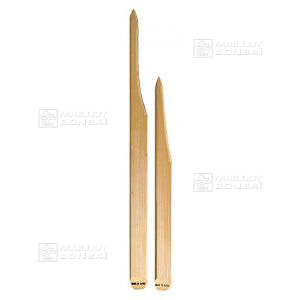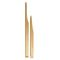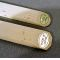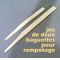##### The Japanese Bonsai specialist
Direct order Contact Help / Services Newsletter Pay in 3 times without charges, from 135 euros in your shopping cart (France residents only)# Bamboo chopsticks 230 and 290mm 2 units

Rempotage 2 baguettes de bambouref. : 4417

4,50

Available quantity : 13Order

###### Description

One is 230 mm long and the other is 290 mm long. In bamboo: 15 mm * 4 mm +-. Sold as a pair. These sticks are for detangling the root system. They allow you to get in between the roots and the soil when repotting.

#bamboo 3.6 #chopsticks 3 #potting 2.7 #units 2.5 #tools 2.5 #long 2.4 #detangling 2 #repotting 1.9 #between 1.7 #sticks 1.6

Formule
(( ROUND((CHAR_LENGTH(b.article_nom)-CHAR_LENGTH(REPLACE(b.article_nom, 'bamboo', '')))/LENGTH('bamboo')) + ROUND((CHAR_LENGTH(b.article_description)-CHAR_LENGTH(REPLACE(b.article_description, 'bamboo', '')))/LENGTH('bamboo')) ) * 3.6) + (( ROUND((CHAR_LENGTH(b.article_nom)-CHAR_LENGTH(REPLACE(b.article_nom, 'chopsticks', '')))/LENGTH('chopsticks')) + ROUND((CHAR_LENGTH(b.article_description)-CHAR_LENGTH(REPLACE(b.article_description, 'chopsticks', '')))/LENGTH('chopsticks')) ) * 3) + (( ROUND((CHAR_LENGTH(b.article_nom)-CHAR_LENGTH(REPLACE(b.article_nom, 'units', '')))/LENGTH('units')) + ROUND((CHAR_LENGTH(b.article_description)-CHAR_LENGTH(REPLACE(b.article_description, 'units', '')))/LENGTH('units')) ) * 2.5) + (( ROUND((CHAR_LENGTH(b.article_nom)-CHAR_LENGTH(REPLACE(b.article_nom, 'long', '')))/LENGTH('long')) + ROUND((CHAR_LENGTH(b.article_description)-CHAR_LENGTH(REPLACE(b.article_description, 'long', '')))/LENGTH('long')) ) * 2.4) + (( ROUND((CHAR_LENGTH(b.article_nom)-CHAR_LENGTH(REPLACE(b.article_nom, 'detangling', '')))/LENGTH('detangling')) + ROUND((CHAR_LENGTH(b.article_description)-CHAR_LENGTH(REPLACE(b.article_description, 'detangling', '')))/LENGTH('detangling')) ) * 2) + (( ROUND((CHAR_LENGTH(b.article_nom)-CHAR_LENGTH(REPLACE(b.article_nom, 'repotting', '')))/LENGTH('repotting')) + ROUND((CHAR_LENGTH(b.article_description)-CHAR_LENGTH(REPLACE(b.article_description, 'repotting', '')))/LENGTH('repotting')) ) * 1.9) + (( ROUND((CHAR_LENGTH(b.article_nom)-CHAR_LENGTH(REPLACE(b.article_nom, 'between', '')))/LENGTH('between')) + ROUND((CHAR_LENGTH(b.article_description)-CHAR_LENGTH(REPLACE(b.article_description, 'between', '')))/LENGTH('between')) ) * 1.7) + (( ROUND((CHAR_LENGTH(b.article_nom)-CHAR_LENGTH(REPLACE(b.article_nom, 'system', '')))/LENGTH('system')) + ROUND((CHAR_LENGTH(b.article_description)-CHAR_LENGTH(REPLACE(b.article_description, 'system', '')))/LENGTH('system')) ) * 1.6) + (( ROUND((CHAR_LENGTH(b.article_nom)-CHAR_LENGTH(REPLACE(b.article_nom, 'sticks', '')))/LENGTH('sticks')) + ROUND((CHAR_LENGTH(b.article_description)-CHAR_LENGTH(REPLACE(b.article_description, 'sticks', '')))/LENGTH('sticks')) ) * 1.6) + (( ROUND((CHAR_LENGTH(b.article_nom)-CHAR_LENGTH(REPLACE(b.article_nom, 'allow', '')))/LENGTH('allow')) + ROUND((CHAR_LENGTH(b.article_description)-CHAR_LENGTH(REPLACE(b.article_description, 'allow', '')))/LENGTH('allow')) ) * 1.5)

## Secure payment## Delivery

Our logistic partners :